# 3 7 Interpret the Quotient Learn to solve

• Slides: 9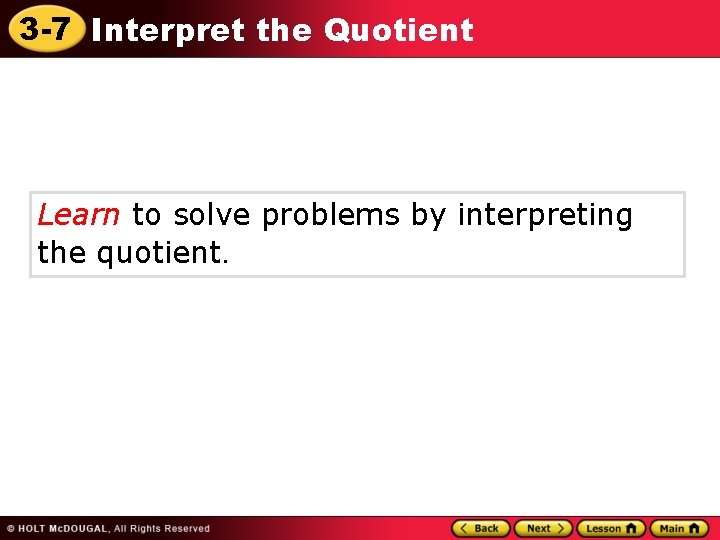3 -7 Interpret the Quotient Learn to solve problems by interpreting the quotient.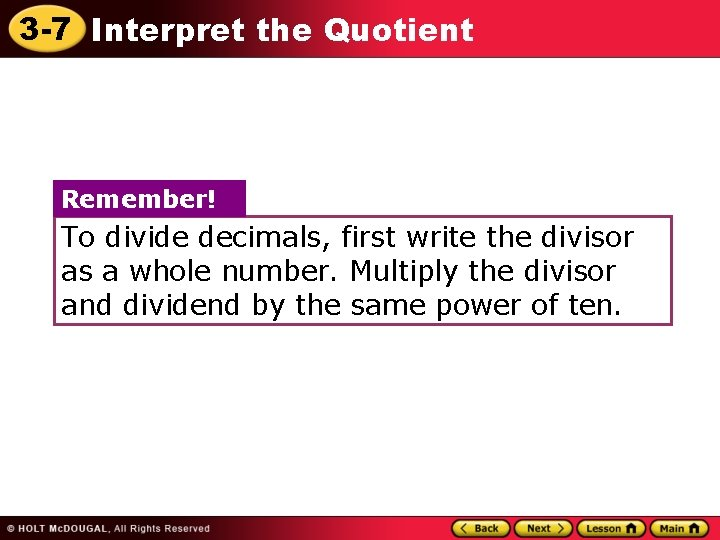3 -7 Interpret the Quotient Remember! To divide decimals, first write the divisor as a whole number. Multiply the divisor and dividend by the same power of ten.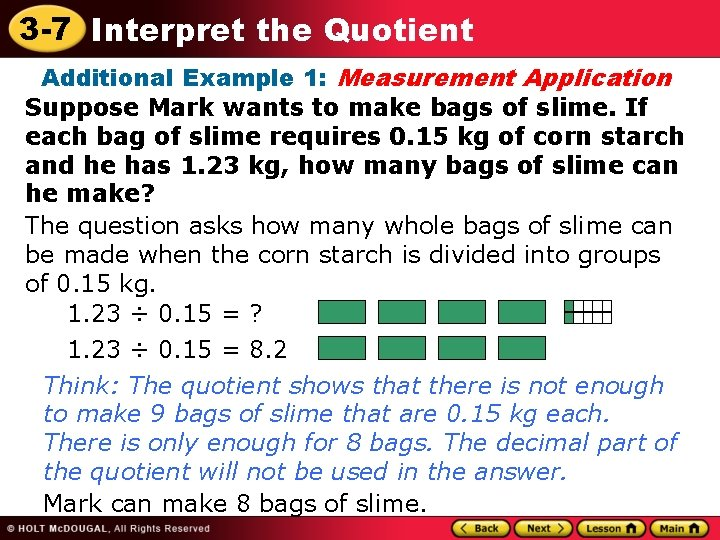3 -7 Interpret the Quotient Additional Example 1: Measurement Application Suppose Mark wants to make bags of slime. If each bag of slime requires 0. 15 kg of corn starch and he has 1. 23 kg, how many bags of slime can he make? The question asks how many whole bags of slime can be made when the corn starch is divided into groups of 0. 15 kg. 1. 23 ÷ 0. 15 = ? 1. 23 ÷ 0. 15 = 8. 2 Think: The quotient shows that there is not enough to make 9 bags of slime that are 0. 15 kg each. There is only enough for 8 bags. The decimal part of the quotient will not be used in the answer. Mark can make 8 bags of slime.3 -7 Interpret the Quotient Check It Out: Example 1 Suppose Antonio wants to make bags of slime. If each bag of slime requires 0. 15 kg of corn starch and he has 1. 44 kg, how many bags of slime can he make? The question asks how many whole bags of slime can be made when the corn starch is divided into groups of 0. 15 kg. 1. 44 ÷ 0. 15 = ? 1. 44 ÷ 0. 15 = 9. 6 Think: The quotient shows that there is not enough to make 10 bags of slime that are 0. 15 kg each. There is only enough for 9 bags. The decimal part of the quotient will not be used in the answer. Antonio can make 9 bags of slime.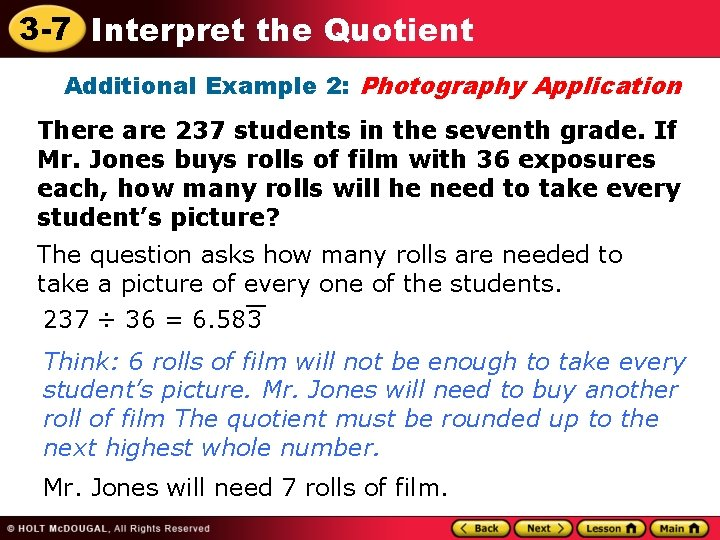3 -7 Interpret the Quotient Additional Example 2: Photography Application There are 237 students in the seventh grade. If Mr. Jones buys rolls of film with 36 exposures each, how many rolls will he need to take every student’s picture? The question asks how many rolls are needed to take a picture of every one of the students. __ 237 ÷ 36 = 6. 583 Think: 6 rolls of film will not be enough to take every student’s picture. Mr. Jones will need to buy another roll of film The quotient must be rounded up to the next highest whole number. Mr. Jones will need 7 rolls of film.3 -7 Interpret the Quotient Check It Out: Example 2 There are 342 students in the seventh grade. If Ms. Tia buys rolls of film with 24 exposures each, how many rolls will she need to take every student’s picture? The question asks how many rolls are needed to take a picture of every one of the students. 342 ÷ 24 = 14. 25 Think: 14 rolls of film will not be enough to take every student’s picture. Ms. Tia will need to buy another roll of film. The quotient must be rounded up to the next highest whole number. Ms. Tia will need 15 rolls of film.3 -7 Interpret the Quotient Additional Example 3: Social Studies Application Gary has 42. 25 meters of rope. If he cuts it into 13 equal pieces, how long is each piece? The question asks how long each piece will be when the rope is cut into 13 pieces. 42. 25 ÷ 13 = 3. 25 Think: The question asks for an exact answer, so do not estimate. Use the entire quotient. Each piece will be 3. 25 meters long.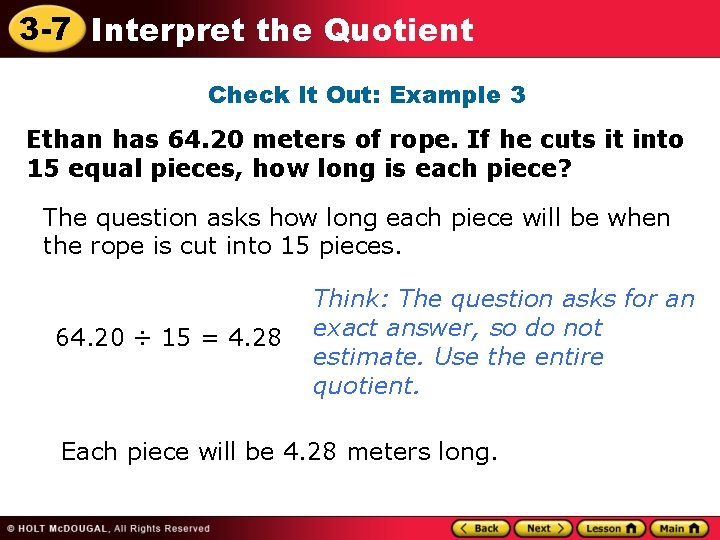3 -7 Interpret the Quotient Check It Out: Example 3 Ethan has 64. 20 meters of rope. If he cuts it into 15 equal pieces, how long is each piece? The question asks how long each piece will be when the rope is cut into 15 pieces. 64. 20 ÷ 15 = 4. 28 Think: The question asks for an exact answer, so do not estimate. Use the entire quotient. Each piece will be 4. 28 meters long.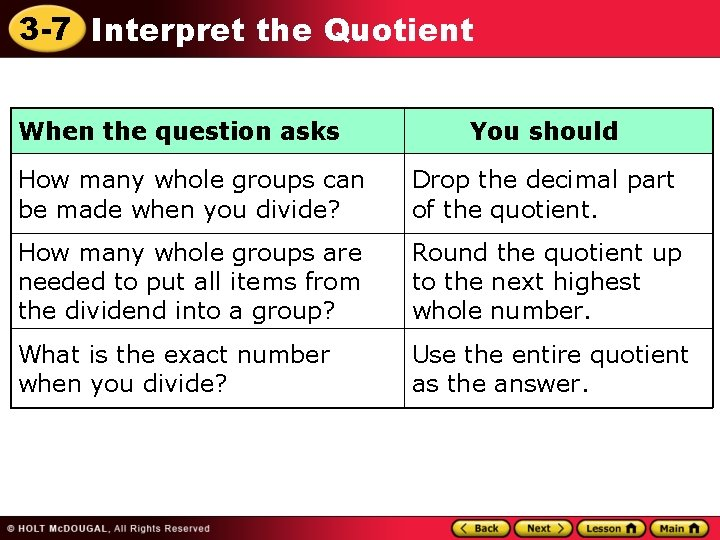3 -7 Interpret the Quotient When the question asks You should How many whole groups can be made when you divide? Drop the decimal part of the quotient. How many whole groups are needed to put all items from the dividend into a group? Round the quotient up to the next highest whole number. What is the exact number when you divide? Use the entire quotient as the answer.